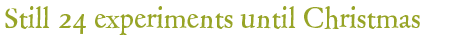## Experiment of December 9, 2022:

The time to submit an answer is over. You can now view the solution of the experiment.

## Experiment:

The following file is needed for the experiment: PiA2022_Experiment_09.pdf.

## Question:

What does Ms. Santa observe?

A) If edge A lies on edge A, the two sheets always lie close together, if edge A lies on edge B, the two sheets diverge in one orientation, if they are turned around they lie on top of each other.

B) If edge A lies on edge A, the two sheets are always close together, if edge A lies on edge B, it is the same.

C) If edge A lies on edge B, the two sheets are always close together, if edge A lies on edge A, the two sheets diverge in one orientation, upside down they lie on top of each other.

D) If edge A lies on edge B, the two sheets diverge in one orientation, upside down they lie on top of each other, if edge A lies on edge A, it is exactly the same.